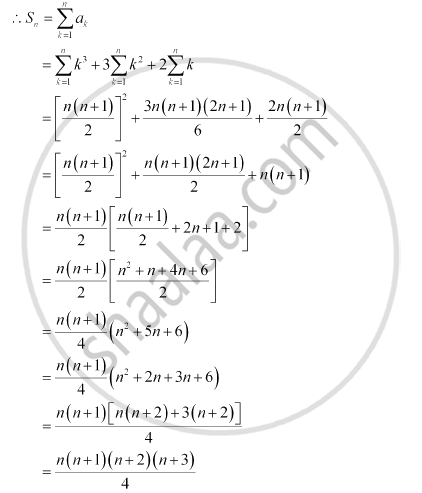# Find the Sum to N Terms of the Series 1 × 2 × 3 + 2 × 3 × 4 + 3 × 4 × 5 + … - Mathematics

Find the sum to n terms of the series 1 × 2 × 3 + 2 × 3 × 4 + 3 × 4 × 5 + …

#### Solution

The given series is 1 × 2 × 3 + 2 × 3 × 4 + 3 × 4 × 5 + …

nth term, an = n ( n + 1) ( n + 2)

= (n2 + n) (n + 2)

= n+ 3n+ 2nConcept: Sum to N Terms of Special Series
Is there an error in this question or solution?

#### APPEARS IN

NCERT Class 11 Mathematics
Chapter 9 Sequences and Series
Q 2 | Page 196